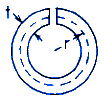Related Resources: calculators

### Thin Circular Open Tube Torsional Deformation and Stress Equation and Calculator

Beam Deflection and Stress Equation and Calculators

Torsional Deformation and Stress Thin Circular Open Tube of Uniform Thickness Section Equations and Calculator.ALL calculators require a Premium Membership

Preview

Torsional Deformation and Stress Hollow Thin Circular Open Tube of Uniform Thickness Calculator

K = (2/3) π r t3

Angle of Twist under applied Torque Moment

θ = ( T L ) / ( K G )

Shear Stress MaximumAlong both edges remote from ends and assumes t is small compared with mean radius.

Where:

θ = angle of twist (radians)
α = degrees
T = Twisting or torque moment force-length, (in-lbs, N-mm)
L = Length (in, mm)
t = Thickness wall (in, mm)
τ = Unit shear stress force / area (lbs/in2, N/mm2)
G = Modulus of rigidity force / area (lbs/in2, N/mm2)
K = Polar Moment of Inertia (in4, mm4) for section

Reference:
Roarks Formulas for Stress and Strain, 7th Edition, Table 10.1 Formulas for torsional deformation and stress.